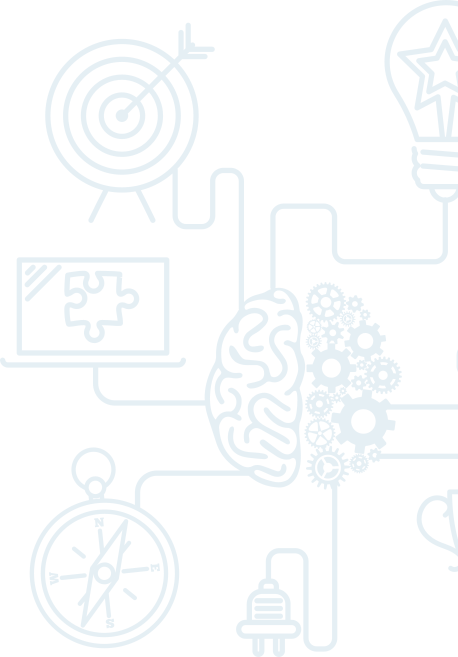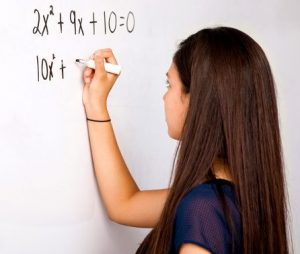# Math Level D (formerly Level 3)

In response to customer feedback, we have expanded and re-levelled our math program for Fall-Winter 2023-24. As a result, some of our courses have been renamed for 2023-24.Our Level D Math & Problem-Solving program (formerly Level 3) typically targets students in Grades 7 through 9. However, younger students may, albeit rarely, be placed at Level D if they meet all prerequisites for this level. This level addresses, in depth, all four steps of problem solving by applying these to quite complex and difficult problems.

Students learn to think abstractly, collaborate with others, persevere, and approach problems from different perspectives.  Lessons include interactive puzzles, brain teasers, logic problems, and competitions to help students stay sharp, discover new strategies, and develop mathematical thinking through numerous approaches.

Level D instruction includes, but is not limited to:

Math

• Numerical systems: Egyptian, Roman, base 2, base 5, and other
• Elements of formal logic (Boolean Algebra)
• Probability
• Equations and systems of equations
• Introduction to matrices
• Difficult problems on percent and proportions
• Application of statistics and measures of centre
• Electrical circuits for simple Boolean operators
• Algebra: complex problems on one variable and on two variables
• Surface area and volume
• Square roots and exponents

Strategy games & logic puzzles

• Riddles
• Crack the code
• Mazes
• Optical illusions
• Mathematical theorems
• Competitions
• Games
• Group presentation
• 3D and visual design

Managing Stress and Test Anxiety

• Time management
• Planning and organization
• Focusing techniques
• Mindfulness

Logic and Problem Solving Strategies

• Reading comprehension and 4-step problem solving
• Understand, model and apply problem solving techniques
• Choose and apply appropriate problem solving strategy:
• Forward solving strategies
• Backwards solving strategies
• Venn diagrams
• Logical trees
• Logical charts
• Systematic list
• Problem decomposition
• Encryption and decoding
• Using and playing formulas
• Modeling with equations and graphs
• Finding and applying patterns
• Translating scenarios into algebraic equations

Prerequisite for Level 3: Knowledge of positive and negative numbers, elementary algebra, and problem-solving strategies and techniques from Level 2; OR successful completion of interview (for new students).